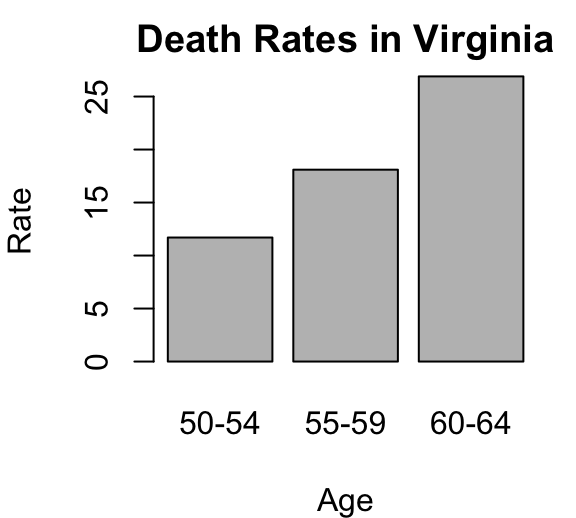# Bar Plots - R Base Graphs

Previously, we described the essentials of R programming and provided quick start guides for importing data into R.

Here, we’ll describe how to create bar plots in R. The function barplot() can be used to create a bar plot with vertical or horizontal bars.

1. Launch RStudio as described here: Running RStudio and setting up your working directory

2. Prepare your data as described here: Best practices for preparing your data and save it in an external .txt tab or .csv files

3. Import your data into R as described here: Fast reading of data from txt|csv files into R: readr package.

Here, we’ll use the R built-in VADeaths data set.

``````# Data set
``````##       Rural Male Rural Female Urban Male Urban Female
## 50-54       11.7          8.7       15.4          8.4
## 55-59       18.1         11.7       24.3         13.6
## 60-64       26.9         20.3       37.0         19.3
## 65-69       41.0         30.9       54.6         35.1
## 70-74       66.0         54.3       71.1         50.0``````
``````# Subset
x``````
``````## 50-54 55-59 60-64
##  11.7  18.1  26.9``````

# Basic bar plots

``````# Bar plot of one variable
barplot(x)
# Horizontal bar plot
barplot(x, horiz = TRUE)``````## Change group names

``barplot(x, names.arg = c("A", "B", "C"))``## Change color

``````# Change border and fill color using one single color
barplot(x, col = "white", border = "steelblue")
# Change the color of border.
#  Use different colors for each group
barplot(x, col = "white",
border = c("#999999", "#E69F00", "#56B4E9"))
# Change fill color : single color
barplot(x, col = "steelblue")
# Change fill color: multiple colors
barplot(x, col = c("#999999", "#E69F00", "#56B4E9"))``````## Change main title and axis labels

``````# Change axis titles
# Change color (col = "gray") and remove frame
barplot(x, main = "Death Rates in Virginia",
xlab = "Age", ylab = "Rate")``````# Stacked bar plots

``````barplot(VADeaths,
col = c("lightblue", "mistyrose", "lightcyan",
"lavender", "cornsilk"),# Grouped bar plots

``````barplot(VADeaths,
col = c("lightblue", "mistyrose", "lightcyan",
"lavender", "cornsilk"),
legend = rownames(VADeaths), beside = TRUE)``````It’s also possible to add legends to a plot using the function legend() as follow.

``````# Define a set of colors
my_colors <- c("lightblue", "mistyrose", "lightcyan",
"lavender", "cornsilk")
# Bar plot
barplot(VADeaths, col = my_colors, beside = TRUE)
fill = my_colors, box.lty = 0, cex = 0.8)``````• box.lty = 0: Remove the box around the legend
• cex = 0.8: legend text size

# Infos

This analysis has been performed using R statistical software (ver. 3.2.4).